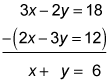##### SAT: 1,001 Practice Questions For DummiesYou will probably encounter some questions on the SAT Math exam that deal with systems of equations. In the following practice questions, you're given the system of equations, and you have to find the value of the variables x and y.

## Practice questions

1. Based on this system of equations, what is the value of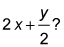7x + 3y = 4 3x + 2y = 2
2. Based on this system of equations, what is the value of x + y?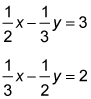1. The correct answer is 1. You don't need separate values of x or y, so don't bother substituting. Simply subtract the equations and divide by 2: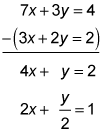2. The correct answer is 6. Subtract the equations: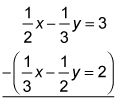A common denominator works, but you could also multiply everything by 6: